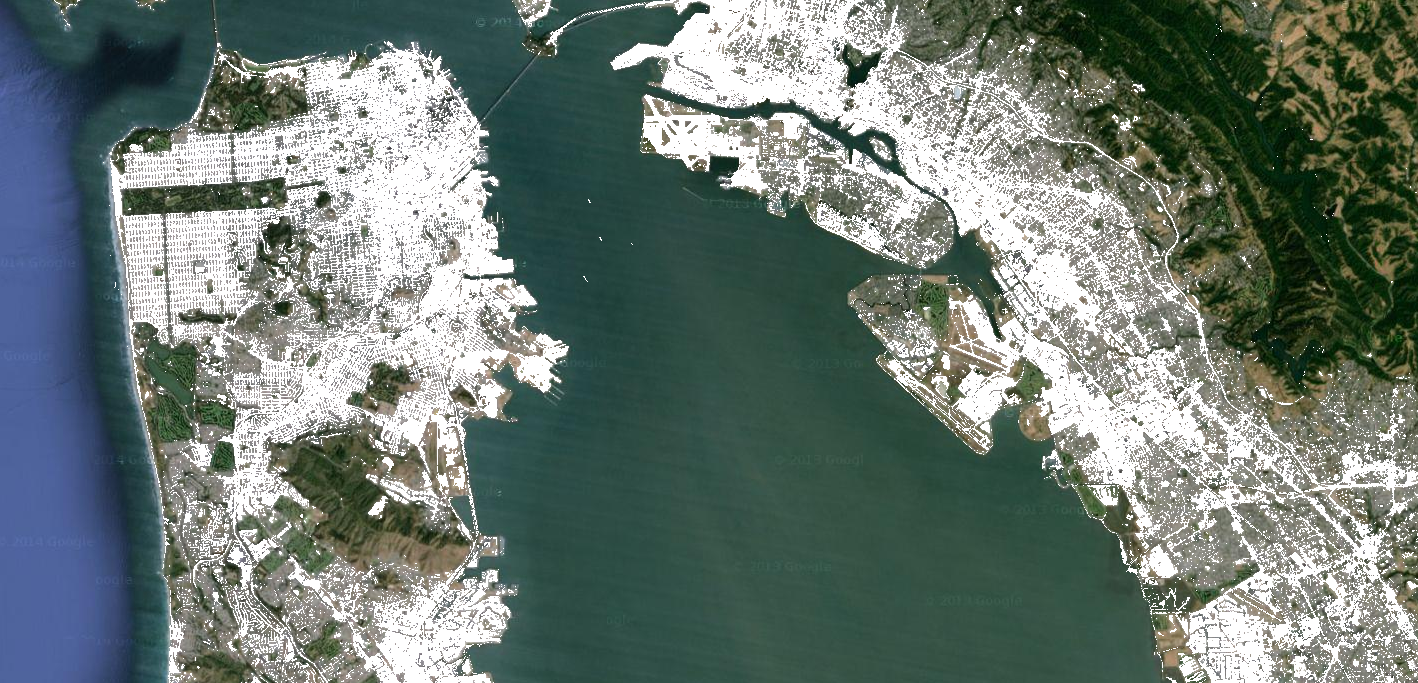# Relational, Conditional, and Boolean Operations

`ee.Image` objects have a set of relational, conditional, and boolean methods for constructing decision-making expressions. The results of these methods are useful for limiting analysis to certain pixels or regions through masking, developing classified maps, and value reassignment.

## Relational and boolean operators

Relational methods include:

`eq()`, `gt()`, `gte()`, `lt()`, and `lte()`

Boolean methods include:

### Code Editor (JavaScript)

`and()`,`or()`, and `not()`

### Colab (Python)

`And()`,`Or()`, and `Not()`

To perform per-pixel comparisons between images, use relational operators. To extract urbanized areas in an image, this example uses relational operators to threshold spectral indices, combining the thresholds with the and operator:

### Code Editor (JavaScript)

```// Load a Landsat 8 image.
var image = ee.Image('LANDSAT/LC08/C02/T1_TOA/LC08_044034_20140318');

// Create NDVI and NDWI spectral indices.
var ndvi = image.normalizedDifference(['B5', 'B4']);
var ndwi = image.normalizedDifference(['B3', 'B5']);

// Create a binary layer using logical operations.
var bare = ndvi.lt(0.2).and(ndwi.lt(0));

// Mask and display the binary layer.
Map.setCenter(-122.3578, 37.7726, 12);
Map.setOptions('satellite');

Python setup

See the Python Environment page for information on the Python API and using `geemap` for interactive development.

```import ee
import geemap.core as geemap```

### Colab (Python)

```# Load a Landsat 8 image.
image = ee.Image('LANDSAT/LC08/C02/T1_TOA/LC08_044034_20140318')

# Create NDVI and NDWI spectral indices.
ndvi = image.normalizedDifference(['B5', 'B4'])
ndwi = image.normalizedDifference(['B3', 'B5'])

# Create a binary layer using logical operations.
bare = ndvi.lt(0.2).And(ndwi.lt(0))

# Define a map centered on San Francisco Bay.
map_bare = geemap.Map(center=[37.7726, -122.3578], zoom=12)

# Add the masked image layer to the map and display it.
display(map_bare)```

As illustrated by this example, the output of relational and boolean operators is either true (1) or false (0). To mask the 0's, you can mask the resultant binary image with itself using `selfMask()`.Low NDVI and low NDWI (white) from Landsat 8, San Francisco, California, USA.

The binary images that are returned by relational and boolean operators can be used with mathematical operators. This example creates zones of urbanization in a nighttime lights image using relational operators and `add()`:

### Code Editor (JavaScript)

```// Load a 2012 nightlights image.
var nl2012 = ee.Image('NOAA/DMSP-OLS/NIGHTTIME_LIGHTS/F182012');
var lights = nl2012.select('stable_lights');

// Define arbitrary thresholds on the 6-bit stable lights band.

// Display the thresholded image as three distinct zones near Paris.
var palette = ['000000', '0000FF', '00FF00', 'FF0000'];
Map.setCenter(2.373, 48.8683, 8);
Map.addLayer(zones, {min: 0, max: 3, palette: palette}, 'development zones');```

Python setup

See the Python Environment page for information on the Python API and using `geemap` for interactive development.

```import ee
import geemap.core as geemap```

### Colab (Python)

```# Load a 2012 nightlights image.
nl_2012 = ee.Image('NOAA/DMSP-OLS/NIGHTTIME_LIGHTS/F182012')
lights = nl_2012.select('stable_lights')

# Define arbitrary thresholds on the 6-bit stable lights band.

# Define a map centered on Paris, France.
map_zones = geemap.Map(center=[48.8683, 2.373], zoom=8)

# Display the thresholded image as three distinct zones near Paris.
palette = ['000000', '0000FF', '00FF00', 'FF0000']
zones, {'min': 0, 'max': 3, 'palette': palette}, 'development zones'
)
display(map_zones)```

## Conditional operators

Note that the code in the previous example is equivalent to using a ternary operator implemented by `expression()`:

### Code Editor (JavaScript)

```// Create zones using an expression, display.
var zonesExp = nl2012.expression(
"(b('stable_lights') > 62) ? 3" +
": (b('stable_lights') > 55) ? 2" +
": (b('stable_lights') > 30) ? 1" +
": 0"
);
{min: 0, max: 3, palette: palette},
'development zones (ternary)');```

Python setup

See the Python Environment page for information on the Python API and using `geemap` for interactive development.

```import ee
import geemap.core as geemap```

### Colab (Python)

```# Create zones using an expression, display.
zones_exp = nl_2012.expression(
"(b('stable_lights') > 62) ? 3 "
": (b('stable_lights') > 55) ? 2 "
": (b('stable_lights') > 30) ? 1 "
': 0'
)

# Define a map centered on Paris, France.
map_zones_exp = geemap.Map(center=[48.8683, 2.373], zoom=8)

# Add the image layer to the map and display it.
zones_exp, {'min': 0, 'max': 3, 'palette': palette}, 'zones exp'
)
display(map_zones_exp)```

Observe that in the previous expression example, the band of interest is referenced using the `b()` function, rather than a dictionary of variable names. Learn more about image expressions on this page. Using either mathematical operators or an expression will produce the same result.

Another way to implement conditional operations on images is with the `where()` operator. Consider the need to replace masked pixels with some other data. In the following example, cloudy pixels are replaced by pixels from a cloud-free image using `where()`:

### Code Editor (JavaScript)

```// Load a cloudy Sentinel-2 image.
var image = ee.Image(
'COPERNICUS/S2_SR/20210114T185729_20210114T185730_T10SEG');
{bands: ['B4', 'B3', 'B2'], min: 0, max: 2000},
'original image');

// Load another image to replace the cloudy pixels.
var replacement = ee.Image(
'COPERNICUS/S2_SR/20210109T185751_20210109T185931_T10SEG');

// Set cloudy pixels (greater than 5% probability) to the other image.
var replaced = image.where(image.select('MSK_CLDPRB').gt(5), replacement);

// Display the result.
Map.setCenter(-122.3769, 37.7349, 11);
{bands: ['B4', 'B3', 'B2'], min: 0, max: 2000},
'clouds replaced');```

Python setup

See the Python Environment page for information on the Python API and using `geemap` for interactive development.

```import ee
import geemap.core as geemap```

### Colab (Python)

```# Load a cloudy Sentinel-2 image.
image = ee.Image('COPERNICUS/S2_SR/20210114T185729_20210114T185730_T10SEG')

# Load another image to replace the cloudy pixels.
replacement = ee.Image(
'COPERNICUS/S2_SR/20210109T185751_20210109T185931_T10SEG'
)

# Set cloudy pixels (greater than 5% probability) to the other image.
replaced = image.where(image.select('MSK_CLDPRB').gt(5), replacement)

# Define a map centered on San Francisco Bay.
map_replaced = geemap.Map(center=[37.7349, -122.3769], zoom=11)

# Display the images on a map.
vis_params = {'bands': ['B4', 'B3', 'B2'], 'min': 0, 'max': 2000}